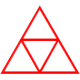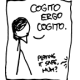# Squares and Octagons

Following is a really fun problem Tom Reardon showed my department last May as he led us through some TI-Nspire CAS training.  Following the introduction of the problem, I offer a mea culpa, a proof, and an extension.

THE PROBLEM:

Take any square and construct midpoints on all four sides.
Connect the four midpoints and four vertices to create a continuous 8-pointed star as shown below.  The interior of the star is an octagon.  Construct this yourself using your choice of dynamic geometry software and vary the size of the square.

Compare the areas of the external square and the internal octagon.You should find that the area of the original square is always 6 times the area of the octagon.

I thought that was pretty cool.  Then I started to play.

MINOR OBSERVATIONS:

Using my Nspire, I measured the sides of the octagon and found it to be equilateral.

As an extension of Tom’s original problem statement, I wondered if the constant square:octagon ratio occurred in any other quadrilaterals.  I found the external quadrilateral was also six times the area of the internal octagon for parallelograms, but not for any more general quadrilaterals.  Tapping my understanding of the quadrilateral hierarchy, that means the property also holds for rectangles and rhombi.

MEA CULPA:

Math teachers always warn students to never, ever assume what they haven’t proven.  Unfortunately, my initial exploration of this problem was significantly hampered by just such an assumption.  I obviously know better (and was reminded afterwards that Tom actually had told us that the octagon was not equiangular–but like many students, I hadn’t listened).   After creating the original octagon, measuring its sides and finding them all equivalent, I errantly assumed the octagon was regular.  That isn’t true.

That false assumption created flaws in my proof and generalizations.  I discovered my error when none of my proof attempts worked out, and I eventually threw everything out and started over.  I knew better than to assume.  But I persevered, discovered my error through back-tracking, and eventually overcame.  That’s what I really hope my students learn.

THE REAL PROOF:

Goal:  Prove that the area of the original square is always 6 times the area of the internal octagon.

Assume the side length of a given square is$2x$, making its area$4x^2$.

The octagon’s area obviously is more complicated.  While it is not regular, the square’s symmetry guarantees that it can be decomposed into four congruent kites in two different ways.  Kite AFGH below is one such kite.Therefore, the area of the octagon is 4 times the area of AFGH.  One way to express the area of any kite is$\frac{1}{2}D_1\cdot D_2$, where$D_1$ and$D_2$ are the kite’s diagonals. If I can determine the lengths of$\overline{AG}$ and$\overline {FH}$, then I will know the area of AFGH and thereby the ratio of the area of the square to the area of the octagon.

The diagonals of every kite are perpendicular, and the diagonal between a kite’s vertices connecting its non-congruent sides is bisected by the kite’s other diagonal.  In terms of AFGH, that means$\overline{AG}$ is the perpendicular bisector of$\overline{FH}$.

The square and octagon are concentric at point A, and point E is the midpoint of$\overline{BC}$, so$\Delta BAC$ is isosceles with vertex A, and$\overline{AE}$ is the perpendicular bisector of$\overline{BC}$.

That makes right triangles$\Delta BEF \sim \Delta BCD$.  Because$\displaystyle BE=\frac{1}{2} BC$, similarity gives$\displaystyle AF=FE=\frac{1}{2} DC=\frac{x}{2}$.  I know one side of the kite.

Let point I be the intersection of the diagonals of AFGH.$\Delta BEA$ is right isosceles, so$\Delta AIF$ is, too, with$m\angle{IAF}=45$ degrees.  With$\displaystyle AF=\frac{x}{2}$, the Pythagorean Theorem gives$\displaystyle IF=\frac{x}{2\sqrt{2}}$.  Point I is the midpoint of$\overline{FH}$, so$\displaystyle FH=\frac{x}{\sqrt{2}}$.  One kite diagonal is accomplished.Construct$\overline{JF} \parallel \overline{BC}$.  Assuming degree angle measures, if$m\angle{FBC}=m\angle{FCB}=\theta$, then$m\angle{GFJ}=\theta$ and$m\angle{AFG}=90-\theta$.  Knowing two angles of$\Delta AGF$ gives the third:$m\angle{AGF}=45+\theta$.I need the length of the kite’s other diagonal,$\overline{AG}$, and the Law of Sines gives$\displaystyle \frac{AG}{sin(90-\theta )}=\frac{\frac{x}{2}}{sin(45+\theta )}$, or$\displaystyle AG=\frac{x \cdot sin(90-\theta )}{2sin(45+\theta )}$.

Expanding using cofunction and angle sum identities gives$\displaystyle AG=\frac{x \cdot sin(90-\theta )}{2sin(45+\theta )}=\frac{x \cdot cos(\theta )}{2 \cdot \left( sin(45)cos(\theta ) +cos(45)sin( \theta) \right)}=\frac{x \cdot cos(\theta )}{\sqrt{2} \cdot \left( cos(\theta ) +sin( \theta) \right)}$

From right$\Delta BCD$, I also know$\displaystyle sin(\theta )=\frac{1}{\sqrt{5}}$ and$\displaystyle cos(\theta)=\frac{2}{\sqrt{5}}$.  Therefore,$\displaystyle AG=\frac{x\sqrt{2}}{3}$, and the kite’s second diagonal is now known.

So, the octagon’s area is four times the kite’s area, or$\displaystyle 4\left( \frac{1}{2} D_1 \cdot D_2 \right) = 2FH \cdot AG = 2 \cdot \frac{x}{\sqrt{2}} \cdot \frac{x\sqrt{2}}{3} = \frac{2}{3}x^2$

Therefore, the ratio of the area of the square to the area of its octagon is$\displaystyle \frac{area_{square}}{area_{octagon}} = \frac{4x^2}{\frac{2}{3}x^2}=6$.

QED

EXTENSIONS:

This was so nice, I reasoned that it couldn’t be an isolated result.

I have extended and proved that the result is true for other modulo-3 stars like the 8-pointed star in the square for any n-gon.  I’ll share that very soon in another post.

I proved the result above, but I wonder if it can be done without resorting to trigonometric identities.  Everything else is simple geometry.   I also wonder if there are other more elegant approaches.

Finally, I assume there are other constant ratios for other modulo stars inside larger n-gons, but I haven’t explored that idea.  Anyone?

### 8 responses to “Squares and Octagons”

1.Tatiana

I have a proof for rectangle/octagon that does not use trig at all (property of centroid and similar triangles). I will check if it works for parallelogram.

2.Five Triangles

We had this problem queued up for our blog, but you beat us to it. Our solution uses “area of a triangle” and not much more:

•chrisharrow

Yours is a MUCH lovelier response. Nice. My solution clearly betrays my serious algebraic bias…

I’m typesetting Tatiana’s response using triangles, similarity, and centroids.

The generalized solution is going to be nice, too.

3.Dave Radcliffe (@daveinstpaul)

Here’s my solution. http://gotmath.com/doc/octagon.pdf

•Simon

Dave, your solution is almost too neat!

•chrisharrow

Dave, I should have replied long ago, but Simon is right … I love the beautiful symmetry of your solution. Thanks for the beautiful contribution.